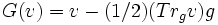Einstein operator

Definition

Given data

A Riemannian manifold$(M,g)$.

Definition part

The Einstein operator$G$ is a map that takes as input a 2-tensor over the Riemannian manifold and outputs another 2-tensor as follows:$G(v) = v - (1/2)(Tr_g v) g$

Note that$G$ maps the Ricci curvature tensor to the Einstein tensor.